Waffle plots are a good way to get amounts across, better than pie charts at least. The most famous example is probably XKCD’s money chart, unfortunately I did not get very close to that using ggplot2 yet.

Here’s three functions that all use a slightly different approach to making waffle plots using ggplot2.

This one uses geom_point, so you can vary the shape.

``````library(formr)
qplot_waffle(rep(1:3,each=40,length.out=90))``````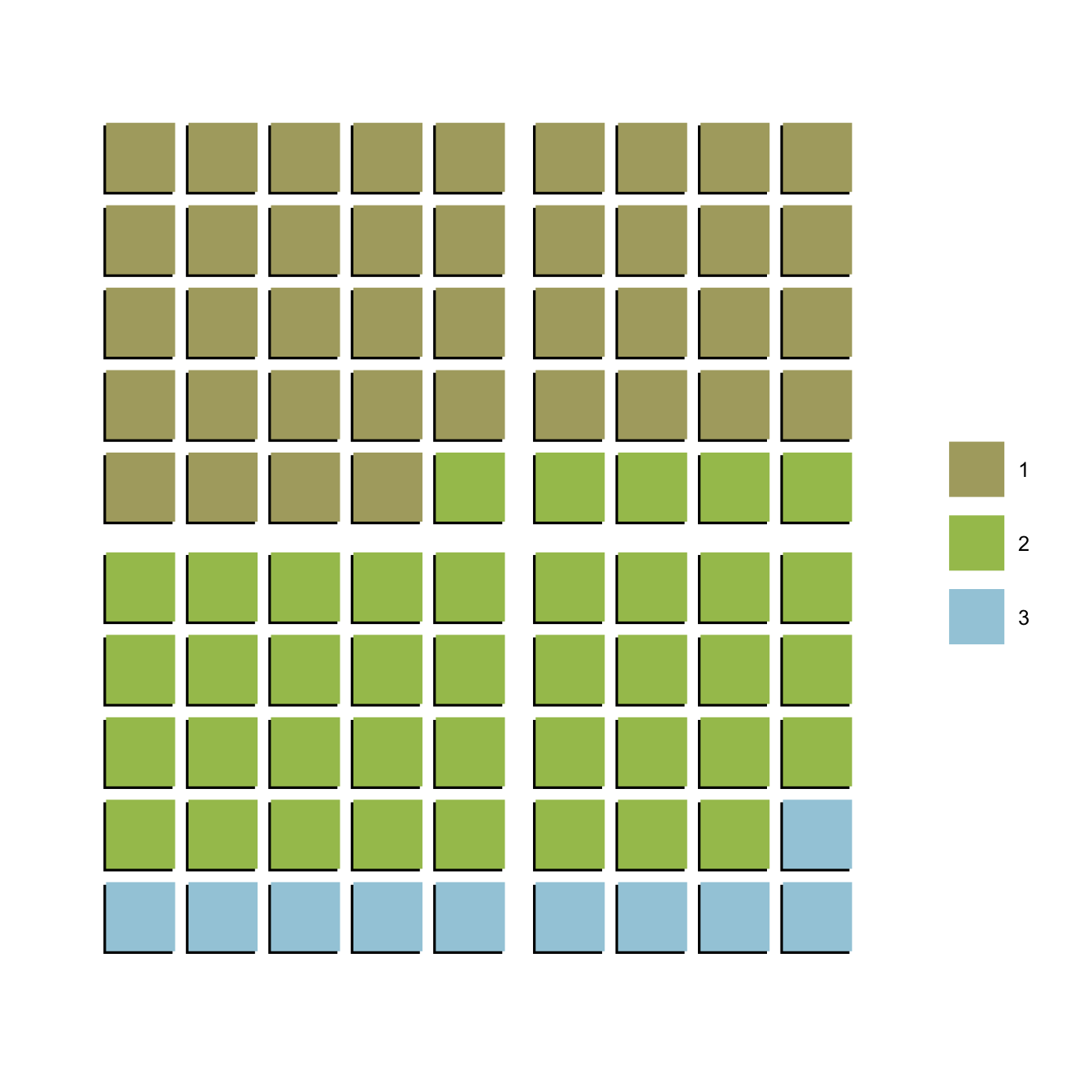This one uses geom_tile. It’s the fastest, but also the least flexible.
This is the only one where the waffle plot still looks reasonable when rescaled a lot. However, here we don’t have drop shadows.

``qplot_waffle_tile(rep(1:2,each=50))``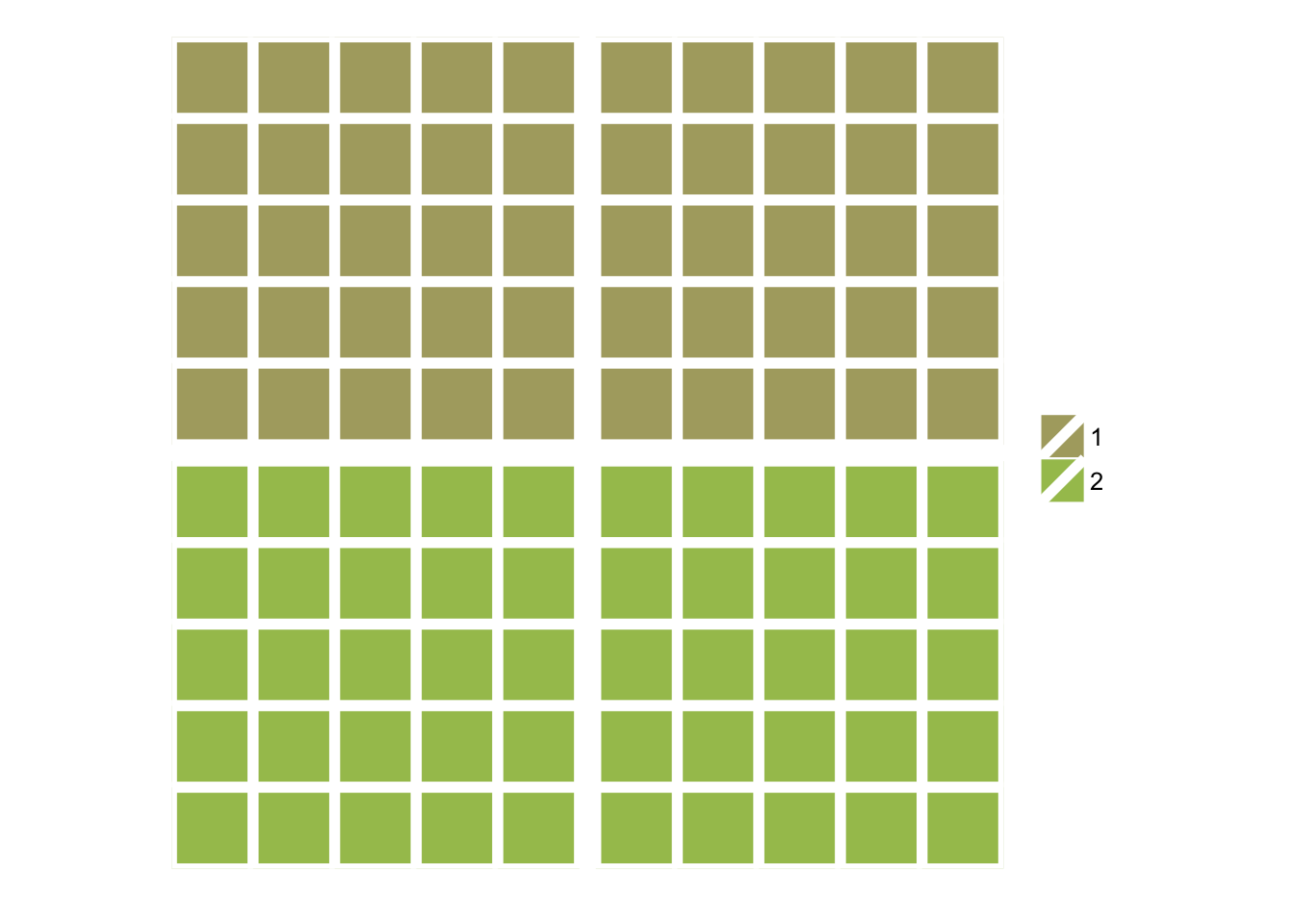This one uses geom_text. This allows us to use custom symbols e.g. from the popular FontAwesome library.

``````library(ggplot2)
qplot_waffle_text(rep(1, each = 30), symbol = "", rows = 3) + ggtitle("Number of travellers in 2008")``````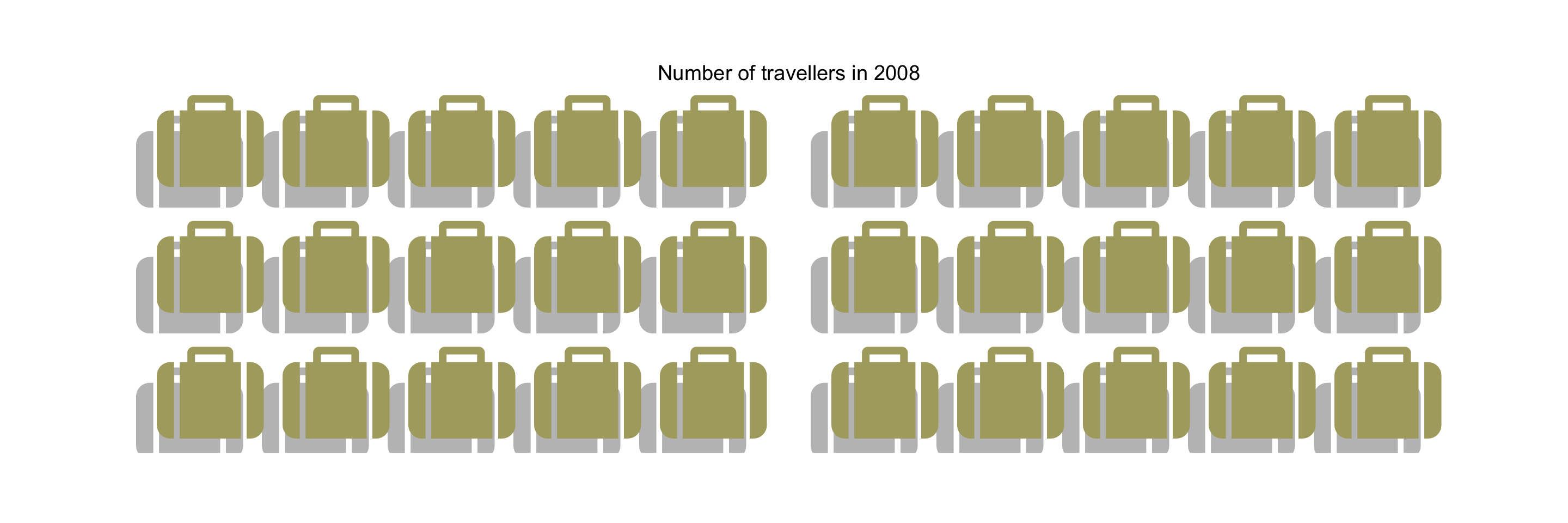Display the data in a smaller unit and add a legend if the amount becomes hard to judge.

``````exports = 1.4e4
qplot_waffle(rep(1,each= 100)) + ggtitle("A hundred dollars") + scale_colour_manual(values="darkgreen")``````
``## Scale for 'colour' is already present. Adding another scale for 'colour', which will replace the existing scale.``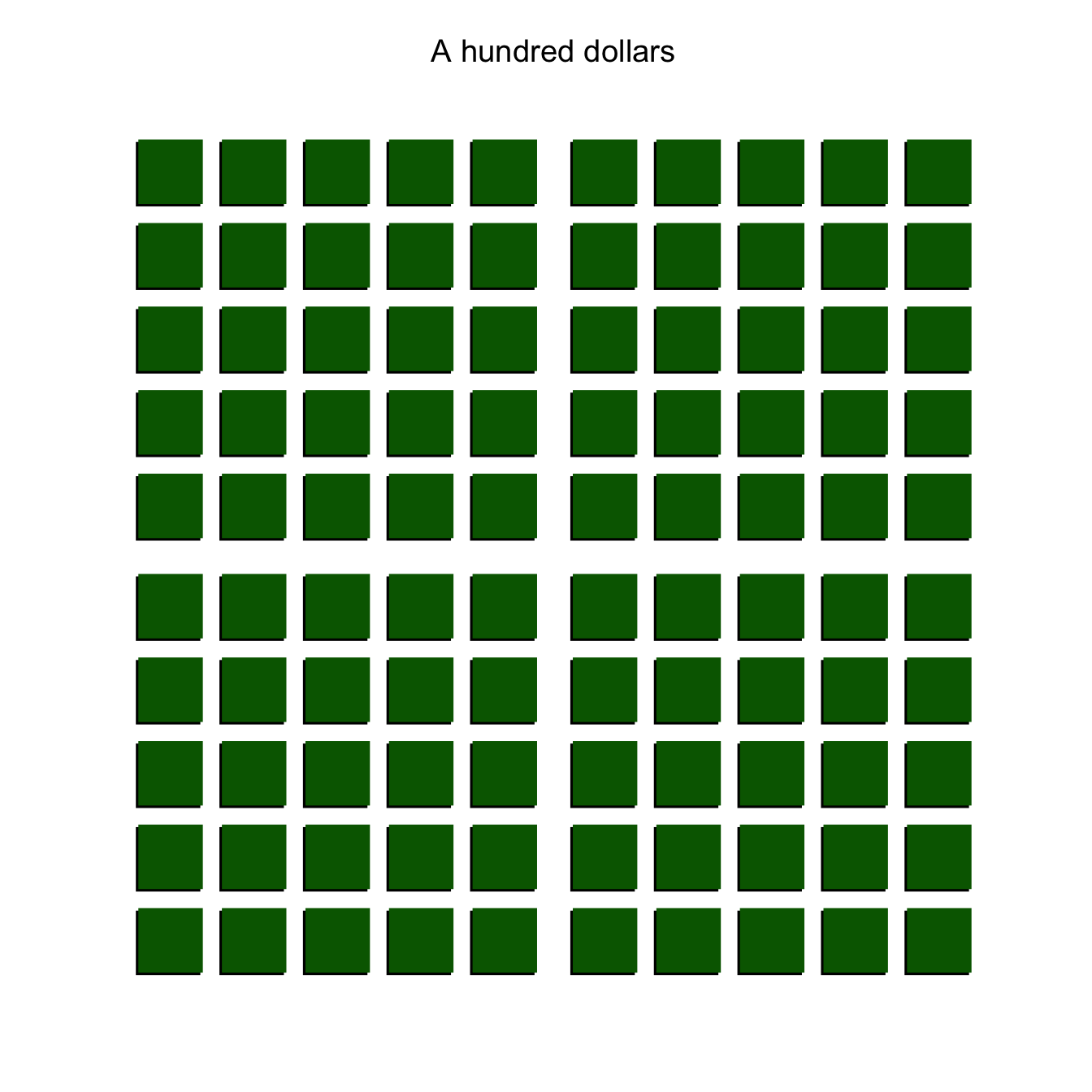``qplot_waffle(rep(1,each= exports/100)) + ggtitle("Sales in hundreds of dollars")``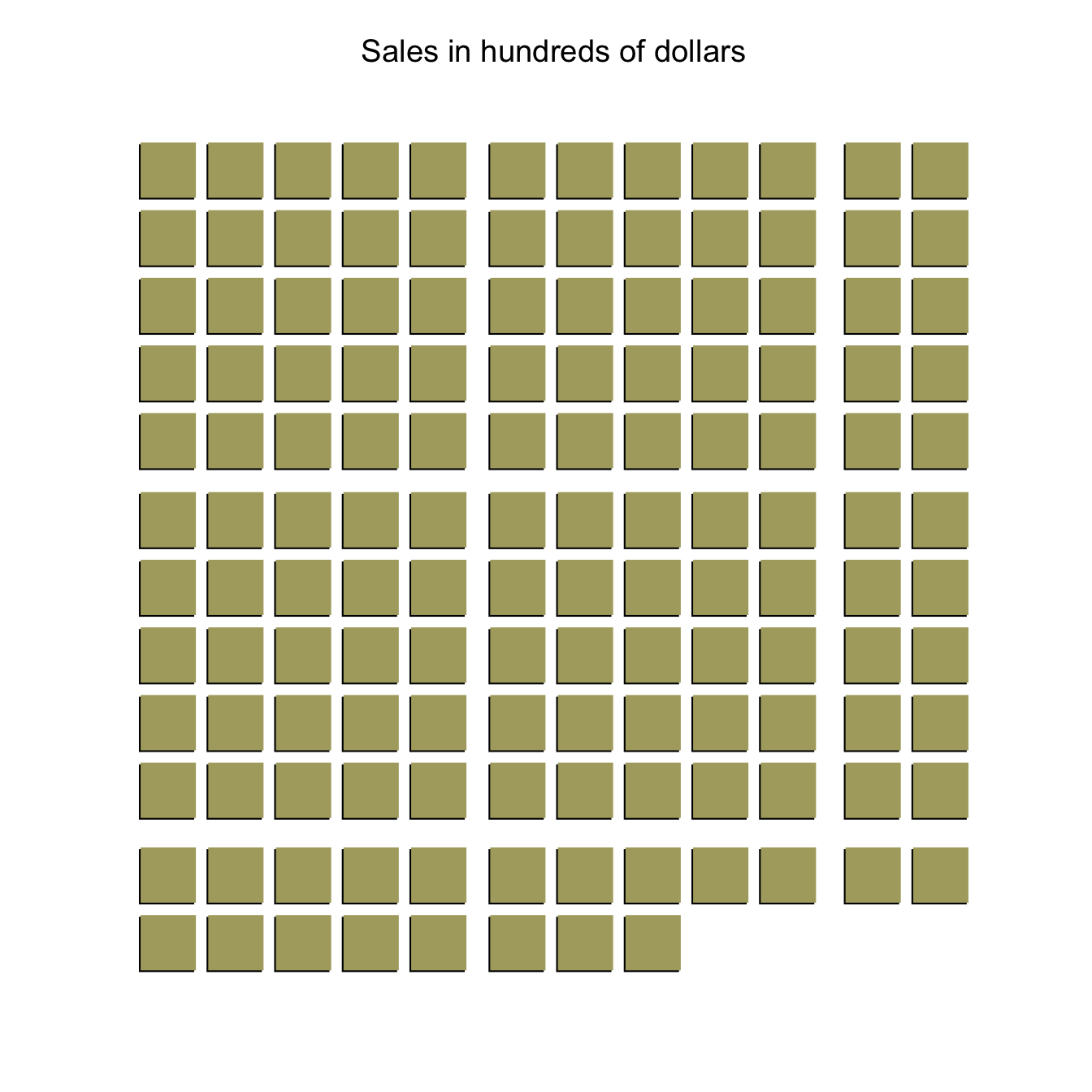Waffle plots don’t use ink sparingly, but can baffle (sorry).

``````library(gridExtra)
to_thousand = function(prop) {
prop = round(prop,2)*1000
c(rep("died", prop), rep("lived to 1y", 1000-prop))
}
old = qplot_waffle_tile(to_thousand(0.128)) + scale_fill_manual(values=c("darkred","gray90")) + ggtitle("Sweden 1737-1900")``````
``## Scale for 'fill' is already present. Adding another scale for 'fill', which will replace the existing scale.``
``modern = qplot_waffle_tile(to_thousand(0.00625999)) + scale_fill_manual(values=c("darkred","gray90"))+ ggtitle("Sweden 1962-1999")``
``## Scale for 'fill' is already present. Adding another scale for 'fill', which will replace the existing scale.``
``grid.arrange(old, modern,main = "Child mortality in Sweden then and now")``